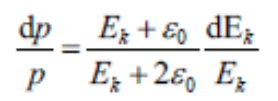# Energy dispersion & Momentum dispersion

Dear FLUKA experts
I am definiting a source and I meet a problem.I wang to add 0.5%Energy dispersion to a 250MeV proton beam but I cannot know how to do it. I find that we definite Momentum dispersion in BEAM card. So I want to know how can I definite Energy dispersion in BEAM card,or I need to transform it to Momentum dispersion?
Thank you very much

Dear @Nicolas_Zhou ,
yes, the use of the BEAM card allows you to define the momentum spread. You have to recalculate energy to the momentum for a given particle rest energy, to set the energy spread value. You can use Flair built in functions T2p(E, p) or directly dT2dp(E, dE, m) to find the value in the Flair built in calculator, or directly in the BEAM card (e.g. set WHAT(2) value =dT2dp(E, dE, m)). If you just recalculate the spread range value you will get asymmetric energy range and distorted energy distribution, as it will be uniform in the momentum, not energy scale. This will be significant for the energy spread higher than 0.5%. In this case, if it is critical for the model, you have to use a SOURCE user routine to define the energy profile programmatically without the Flair interface.

Dear @illia.zymak
I don’t know where to find thers build in functions, could you please tell me where I can find them?
And in my model, I need to compare the Bragg peak (Energy Spread=0.5%) with the Bragg peak(Energy Spread=0).I can not understand you say “it will be uniform in the momentum, not energy scale”, do you mean by the formula